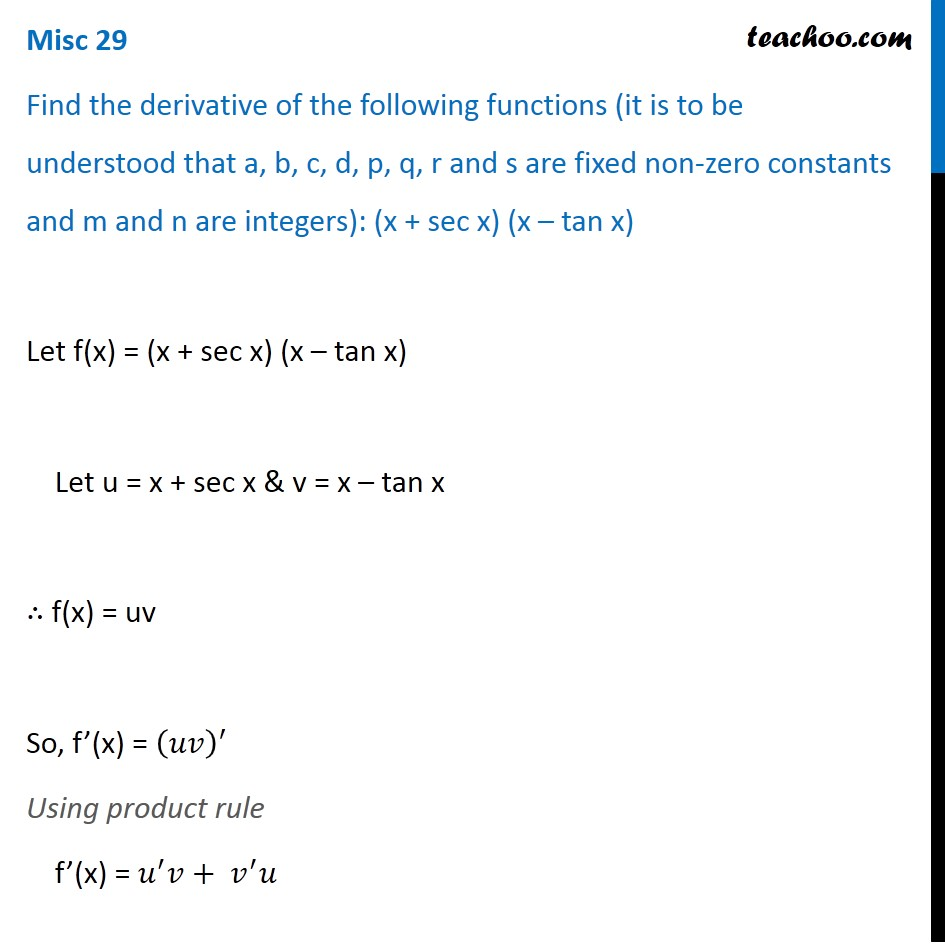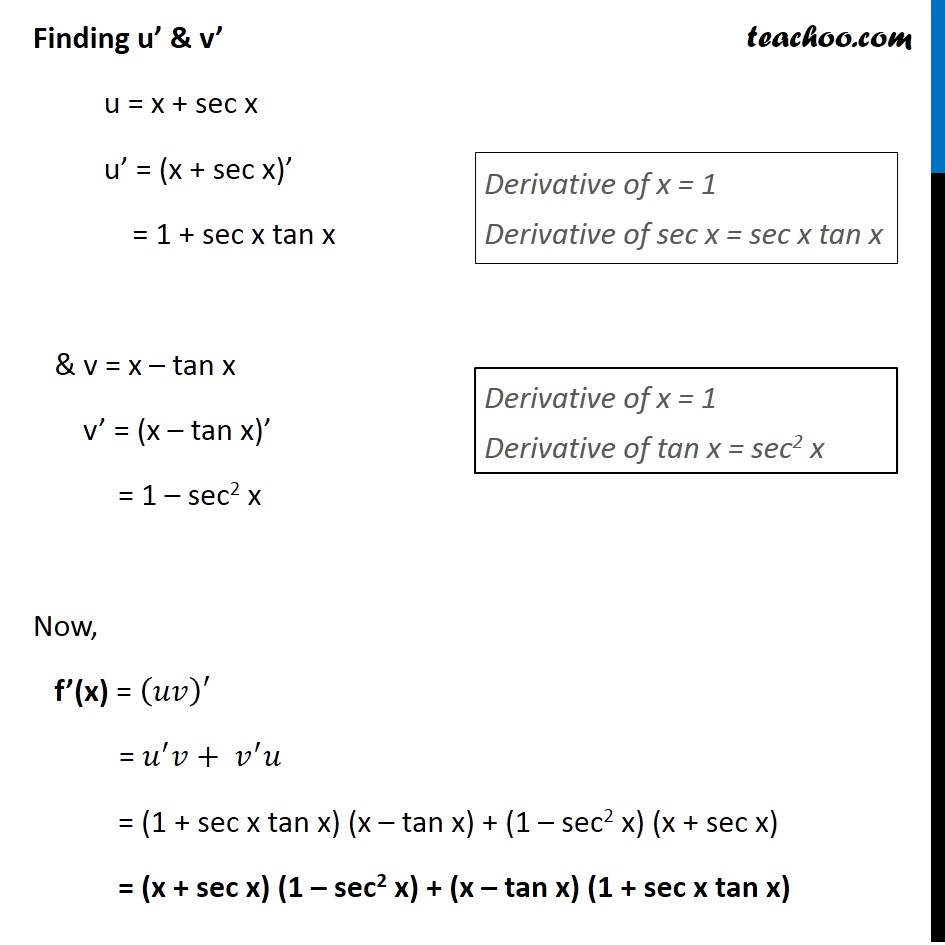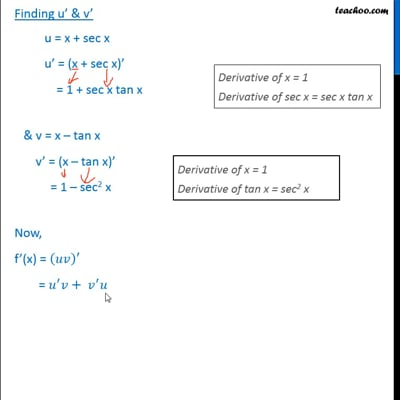Miscellaneous (Term 1 and Term 2)

Chapter 13 Class 11 Limits and Derivatives (Term 1 and Term 2)
Serial order wiseThis video is only available for Teachoo black users

### Transcript

Misc 29 Find the derivative of the following functions (it is to be understood that a, b, c, d, p, q, r and s are fixed non-zero constants and m and n are integers): (x + sec x) (x – tan x) Let f(x) = (x + sec x) (x – tan x) Let u = x + sec x & v = x – tan x ∴ f(x) = uv So, f’(x) = (𝑢𝑣)^′ Using product rule f’(x) = 𝑢^′ 𝑣+〖 𝑣〗^′ 𝑢 Finding u’ & v’ u = x + sec x u’ = (x + sec x)’ = 1 + sec x tan x & v = x – tan x v’ = (x – tan x)’ = 1 – sec2 x Now, f’(x) = (𝑢𝑣)′ = 𝑢^′ 𝑣+〖 𝑣〗^′ 𝑢 = (1 + sec x tan x) (x – tan x) + (1 – sec2 x) (x + sec x) = (x + sec x) (1 – sec2 x) + (x – tan x) (1 + sec x tan x) Derivative of x = 1 Derivative of sec x = sec x tan x Derivative of x = 1 Derivative of tan x = sec2 x